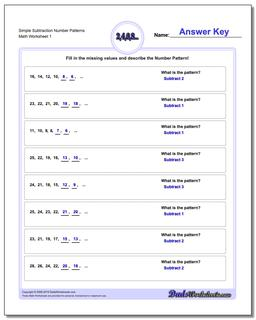# Math Worksheets: Number Patterns: Number Patterns: Simple Subtraction Number Patterns## Simple Subtraction Number Patterns

PropertyValue
DescriptionSimple Subtraction Number Patterns: Number pattern problems using only subtraction operations.
Resource TypeWorksheet